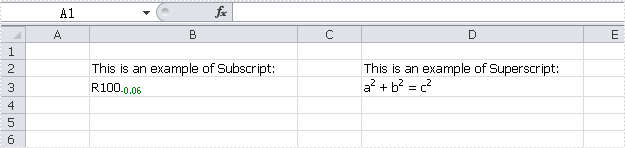# How to Apply Subscript and Superscript in Excel file?

Subscript and superscript are commonly used when dealing with Excel files. Spire.XLS specially designed for developers enables you to apply subscript and superscript in Excel file easily. In this article, a solution is introduced to show how to apply subscript and superscript in Excel file using Spire.XLS.

Step 1: Set the rtf value of "B3" to "R100-0.06"

```CellRange range = sheet.Range["B3"];
range.RichText.Text = "R100-0.06";
```

Step 2: Create a font. Set the IsSubscript property of the font to "true". This font can be used to apply subscript.

```ExcelFont font = workbook.CreateFont();
font.IsSubscript = true;
```

Step 3: Set font for specified range of the text in "B3". In this example, "-0.06" will be formatted as subscript.

```range.RichText.SetFont(4, 8, font);
```

Step 4: Set the rtf value of "D3" to "a2 + b2 = c2".

```range = sheet.Range["D3"];
range.RichText.Text = "a2 + b2 = c2";
```

Step 5: Create a font. Set the IsSuperscript property of the font to "true". This font can be used to apply superscript.

```font = workbook.CreateFont();
font.IsSuperscript = true;
```

Step 6: Set font for specified range of the text in "D3". In this example, "2" will be formatted as superscript.

```range.RichText.SetFont(5, 8, font);
```

Full code and screenshots:

```using Spire.Xls;
using System.Drawing;
namespace ApplySubscriptandSuperscript
{
class Program
{

static void Main(string[] args)
{

Workbook workbook = new Workbook();
Worksheet sheet = workbook.Worksheets;

sheet.Range["B2"].Text = "This is an example of Subscript:";
sheet.Range["D2"].Text = "This is an example of Superscript:";

CellRange range = sheet.Range["B3"];
range.RichText.Text = "R100-0.06";

ExcelFont font = workbook.CreateFont();
font.IsSubscript = true;
font.Color = Color.Green;
range.RichText.SetFont(4, 8, font);

range = sheet.Range["D3"];
range.RichText.Text = "a2 + b2 = c2";

font = workbook.CreateFont();
font.IsSuperscript = true;
range.RichText.SetFont(1, 1, font);
range.RichText.SetFont(6, 6, font);
range.RichText.SetFont(11, 11, font);

sheet.AllocatedRange.AutoFitColumns();
workbook.SaveToFile("result.xls");

}
}
```